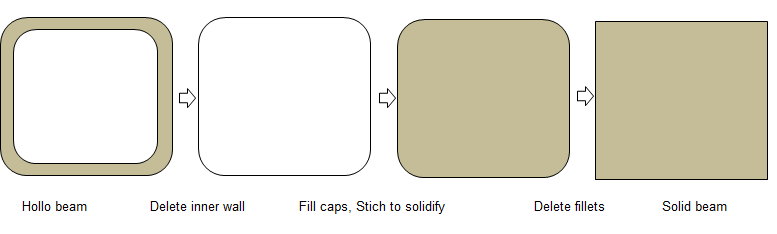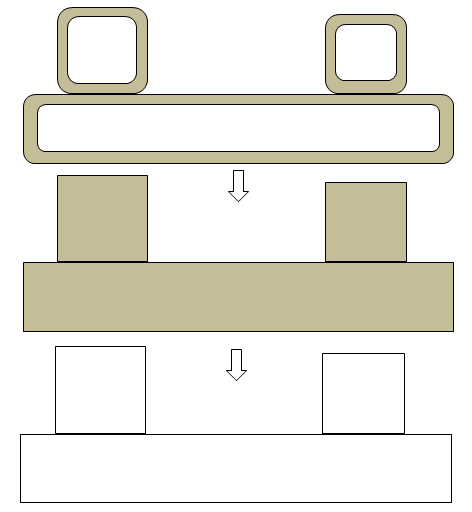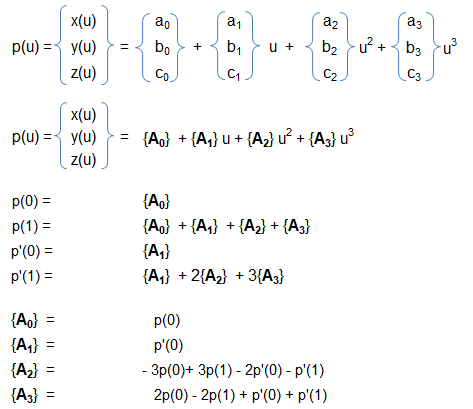• CFD, Fluid Flow, FEA, Heat/Mass Transfer

## Solution Domain

Geometry or computational domain for CFD simulation requires a water tight geometry with simplification to handle the mesh creation process. Solution of computational domain refers to 2D or 3D geometry in which fluid flow and/or heat transfer phenomena need to be solved. This is known as "ZONES" in FLUENT, "REGIONS" in STAR-CCM+ and "DOMAIN" in CFX. These are "volumes surrounded by closed surfaces(called boundaries)" in 3D and "areas closed by edges or lines (called boundaries)" in 2D. It is the mesh and nodes generated to define and represent the physical space mathematically. No geometrical information is associated with the solution domain.

The geometry creation for CFD can be accomplished by either of the following two methods:

• Create geometry in any CAD (Computer-Aided Design) software such as Unigraphics, Catia, Pro/E, Solidedge. The geometry information created so far is called to be in "Native CAD" format. This geometry should be translated to a Neutral format such as IGES, STEP, Parasolid. All Pre-processors available has build-in capacity to read (Import) CAD data in this format. Once the geometry is imported into the Pre-processor, defeature as per requirement.
• The second approach is to create geometry directly into the pre-processor. All commercial pre-processors has basic built-in features to create Geometrical Entities. We strongly recommend this approach for following two cases:
• 2D simulation
• When geometry is axi-symmetric. In this case, one needs to create the 2D cross-section first and then extrude it by rotation. In ANSYS FLUENT, in the axisymmetric model the boundary conditions should be such that the centerline (where the radius reduces to zero) is an axis type instead of a symmetry type. The axis should the z-axis. Thus, for all 2D simulations, FLUENT expects geometry to be in XY-plane.
• One widely used term in numerical simulation is Topology. A precise explanation is provided by Georgios Balafas in his master's thesis for the Master of Science program in Computational Mechanics "Polyhedral Mesh Generation for CFD-Analysis of Complex Structures": The topology of a mesh is an abstraction of the geometric model, that provides unambiguous, shape independent information about the relation between the entities that form the mesh structure. To this end, a mesh database is used in order to store various-level attributes of entities of different dimensions. These typically include 0-D entities, referred to, in literature, as vertices or nodes, 1-D entities, referred to as edges, 2-D entities, referred to as faces or facets and 3-D entities, referred to as solids, volumes, regions or cells.
• Few other definition taken from previous reference:
• "A face is said to be classified on the boundary of the geometric model, when it is adjacent to only one solid, which means that the free side of the face forms an external boundary. In the opposite case, where a face is adjacent to two solids, it is classified in the interior of the geometric model."
• Mesh edges inherit their classification by their adjacent faces. Specifically, when an edge has at least one adjacent face that is classified on a model's boundary surface, the edge is also classified on the same boundary. To be more precise, in a conforming non-manifold three-dimensional mesh, an edge will have either zero or at least 2 adjacent boundary faces, however detecting one of them can be considered enough to classify the edge on the boundary as well. Furthermore, when an edge's adjacent boundary faces are classified on different boundary surfaces of the model, this denotes the edge's classification on a model's boundary edge, where 2 of its boundary surfaces intersect. It is, therefore, adequate to count the amount of different boundary identifiers that are assigned to the adjacent faces of an edge, in order to conclude whether this edge is internal (zero boundary identifiers), on a boundary surface (one identifier), on a boundary edge (2 identifiers), or is a non-manifold edge (> 2 identifiers).
• Extending the aforementioned classification criteria for vertices, a mesh vertex is classified on a model's boundary surface, when at least one of its adjacent edges is also classified on a boundary surface. Additionally, when at least one of the adjacent edges of a vertex is classified on a model's boundary edge, then the vertex is also classified on the same boundary edge. Finally, when there exist at least two adjacent edges classified on two different model's boundary edges, the vertex is classified on a model's corner, formed at the intersection of the boundary edges. In other words, the classification of a vertex with respect to the model can be determined by counting the amount of different boundary identifiers assigned to the adjacent faces of the vertex. Zero boundary identifiers denote an internal vertex, while vertices on a boundary surface have adjacent faces with a unique identifier. Nodes on model's boundary edges have adjacency relationships to faces of two boundary identifiers in total and vertices on a model's corner sum up with three adjacent boundary identifiers. Finally, when a vertex has adjacent faces classified to more than three different boundary surfaces, the vertex is non-manifold.

DOMAIN SIZE REDUCTION THROUGH SIMILARITY: In order to results of the model to apply to the prototype, strict similarity rules must be followed.

A convenient frame of reference must be defined that applies to both model and prototype and corresponding locations must be defined using dimensionless ratios (for a sphere for example, angle on its surface, longitude and latitude lines). Thus, there exist similar conditions for corresponding points on both model and prototype. That is, the drag coefficient at a particular point on the sphere applies to both model and prototype.

The designer must follow, then geometric similarity (model and prototype), kinematic similarity (velocity vector direction is similar for both model and prototype), dynamic similarity (force vector direction is similar for both model and prototype), thermal similarity (heat fluxes), etc.

Note that similarity principles cannot be applied for all application without loss of accuracy. For example, to reduce size of an axial flow fan with shroud, either for numerical or experimental investigation, their is no thumb rule to account for blade-tip and shroud clearance. Hence, the designer has to establish his own "quality standards and acceptance criteria" to address this limitation.

The pre-processing activities require geometry simplifications (defeaturing) and ANSYS SpaceClaim has some powerful non-parametric features. One of them is the 'Pull' option (short-cut 'p') which is equivalent to 'sweep' or 'extrude' operation. By default, pull direction is tangent to the selected edge or perpendicular to the selected face. A direction can be chosen or pulled (sweep) along a curve. Selection: all holes equalt to selected radius, all holes of same radius in same face, all holes of radius equal or smaller than select radius. Blend (b): create surface by two lines (it is equivalent to 'loft' operation in some CAD programs). Fill (f): create surface from a closed loop of curves. Move (m): the 'origin' option is to select the reference point on the source object.

Step-by-Step for Geometry Defeaturing and Simplifications (ANSYS Spaceclaim)

Step-1:

Split the large assemblies into smaller (if possible, geometrically less-dependent) domain. This helps avoid large memory requirement and further geometry clean-up operations as well.

Step-2: Re-group and rename the data

The geometry in CAD environment is created keeping in mind the physical construction and assembly sequences. This is not important in mesh generation. It is better to re-group the geometrical (CAD) entities in terms of expected boundry definition. This makes it easy to operate on a smaller section of the entire domain. The selection, hide/unhide and operations such as imprint/ interference become faster.

Step-3:

Further segregate the geometry into 'Solids' and 'Surfaces'. This helps chose operations appropriate for the respective category. Such as fillet removal, split, booleans and extrude (pull) is easy on solids.

Step-4:

Convert the solids with thin wall thickness into solids with no internal wall and void. For example, a beam with fillets and internal wall.Step-5:

Before removing the wall thicknesss, use the operations appropriate for solids such as Booleans like unite, intersect... to make sure the once converted to surface, no further operations such as extend /intersectio is needed to remove the gaps / connect the surfaces. Note that this method is no recommended for FEA simulation. in CFD the blockage of flow is important where as in FEA the location of centroid is important.Step-5:

ANSYS Spaceclaim is a CAD-type pre-processor and works better on solids. CFD volume extraction is a analogous to 'negative' of visible solid volumes. Instead of deleted the unwanted surfaces one-by-one, it is recommended and worth trying to create a "bounding box" enclosing the geometry and use a bolleaan to subtract the solids from the 'bounding' box. The resultant negative volume of fluid domain is easy to operate and remove the protrusions generated due to gaps in original (input) geometry.
Tips and Tricks:

Definition: gaps in the plane of a surface is called lateral (horizontal) gaps and gaps in the direction of area normal is called the transverse (vertical) gap.

If you are dealing with large surfaces with small (transverse) gaps (say < 1% of the biggest geometry or say 1-5 [mm] which you can neglect), follow these steps:

• Create a plane and/or planar surface encompassing the entire geometry which defines the best possible horizontal plane for the lower bound of the geometry.
• Extend (using 'extrude', 'sweep' or 'pull' tool) by amount which covers (fills) all the unevenness (transverse gaps) in the geometry.
• Split the bodies (solid volumes and surfaces) as per appropriate tool. E.g. in ANSYS Spaceclaim, "Split Body" can be used for both solid and surface bodies. 'Split' tool can be used for surfaces only.
• This process will remove the 'local' details such as 'openings', small surfaces or patches for named selection... Project the curves from original geometry as needed and then split the bigger surfaces created during previous operations.
• Delete the unwanted extensions created due to 'extend' and 'trim / split' operations.
• Repeat the process for other bodies in the assembly.
• Repeat the process for the upper and left / right bounds of the geometry. Though, once the lower and upper bounds are flattened (made coplaner), the 'blend' and 'fill' operation can be used to fill any lateral gaps between vertial edges and surfaces.

Fill operation: selecting many contiguous (connected) surfaces for fill operation does not work. However, selecting just one surface out of many makes the 'fill' tool act nicely.

Do not 'stitch' the geometry body and surfaces until you are dones with all necessary clean-up and simplifications.

'Imprint' and 'Interference' operations do not make intended change in on go. Continue the operation till you get a message "No problem areas were fixed".

In ANSYS Spaceclaim, sometimes 'pull to' operation does not work. However, you can manually 'pull' or 'extend' the edges to or beyond the desired target edge. Hence, extend the edges manually and split them later to have a common boundary.

##### Curves
A curve or segement of a curve is defined as collection of points whose coordinates are specified as continuous ONE-parameter and single-valued mathematical functions. In cartesian coordinate system, it can be expressed as x = f(u), y = g(u) and z = h(u) where f, g and h are the single valued mathematical functions. 'u' is known as parametric variable and it is very often (and necessarily) constrained to the interval [0 1]. The curves are said to be point-bounded as it has two definite end points.

Linear parametric equation of curves: x = a + b.u, y = m + n.u, z = p + q.u where a, b, m, n, p and q are constants. The curves starts at p(u=0) = [a m p] and ends as [(a + b) (m + n) (p + q)] with direction cosines proportional to b, n and q.

Cubical parabola: x = a.u, y = b.u2, z = c.u3 where a, b and c are constants.

Left-handed circular helix: Also known as machine screw, the curves are described as x = r.cos(u), y = r.sin(u), z = p.u where r and p are constants. It is the locus (path) of a point that revolves around the z-axis at radius r and moves parallel to the z-ais at a rate proportional to the angle of revolution 'u' (known as pitch of the helix). Note that if p < 0 then it is a right-handed screw (helix).

All the 3 examples above demonstrate the versatility of parametric representation of a curve. However, we need to always have a independent variable (u or θ) as parameter. Even, x or y or z themselves can be a parameter so that x=f(x), y=g(x) and z=h(x). Similarly, the surfaces can be represented parmetrically. This is the reason the 3D CAD geometry in SolidWorks, Unigraphics, Creo, Inventor, FreeCAD... are called parametric models and the method is called parametric modeling.

Algebraic Form of a Parametric Cubic (PC) Curve: Following 3 polynomials are required to define any curve segment of a PC curve:

x(u) = a0 + a1u + a2u2 + a3u3

y(u) = b0 + b1u + b2u2 + b3u3

z(u) = c0 + c1u + c2u2 + c3u3

The unique set of 12 constant coefficients are known as algebraic coefficients and determines the size, shape and position of the curve in space.

Geometric Form of a Parametric Cubic (PC) Curve: Algebraic coefficients do not lend much flexibility to control the shape of a curve in modeling situations as they do not account for conditions as its ends points or boundaries. When a curve is described based on its conditions as the ends (such as end point coordinates, tangents, curvature, torsion...), it is called geometric form of the curve.Finally, p(u) = (2u3 - 3 u2 + 1) p(0) + (-2u3 + 3u2) p(1) + (u3 - 2u2 + u) p'(0) + (u3 - u2) p'(1).
##### STEP/IGES
STEP (or STP) and IGES (or IGS) are two widely used neutral file format. IGES format was deeloped in 1980 by US Air Force in collaboration with Boeing, GE and Xerox. STEP format came into existence in 1984 as ISO standard 10303-21. Both these standards are plain text formats that use pre-defined special purpose syntax. For example, entity names in STEP format consists of digits preceded by '#'. /* ... */ defines comment. STEP format does not maintain features such as fillets, chamfers... as separate geometrical entities.

The content on CFDyna.com is being constantly refined and improvised with on-the-job experience, testing, and training. Examples might be simplified to improve insight into the physics and basic understanding. Linked pages, articles, references, and examples are constantly reviewed to reduce errors, but we cannot warrant full correctness of all content.